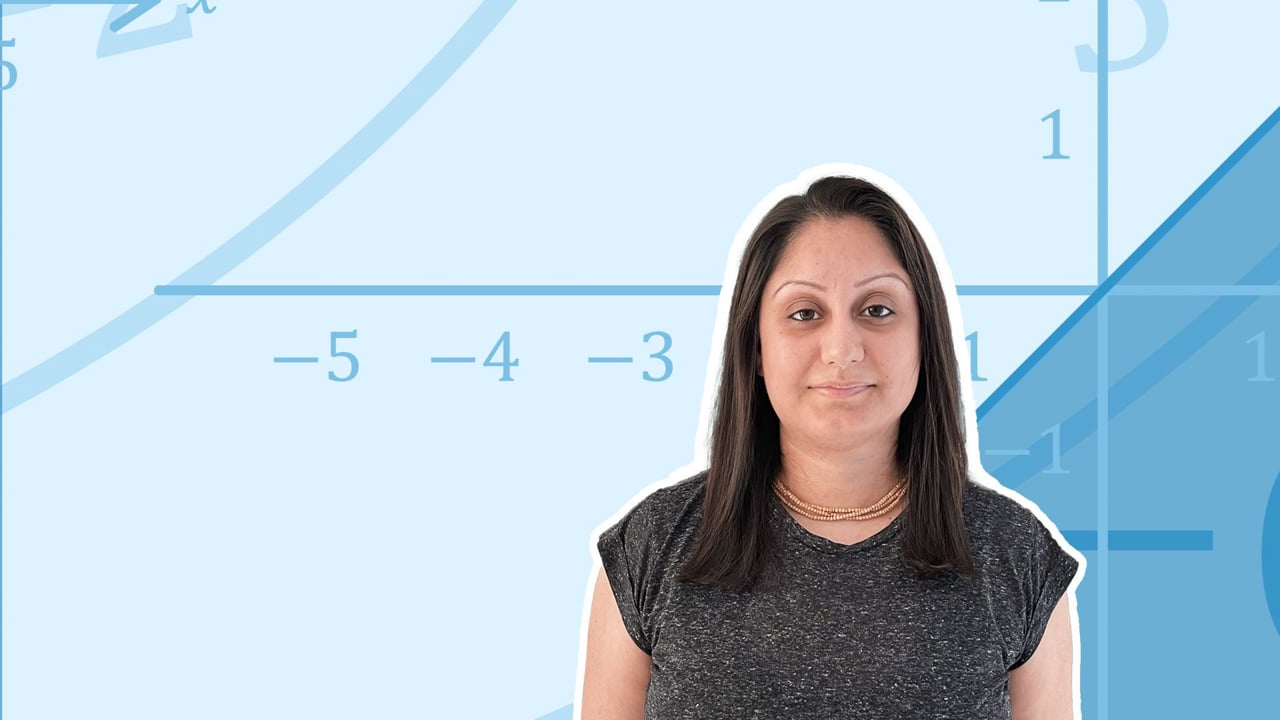Chapter overviewMaths

Exam board

AQA

Number

Algebra

Graphs

Ratio proportion and rates of change

Shapes and area

Angles and geometry

Trigonometry

Probability

Statistics

Maths

# Simplifying algebraic expressions0%

Summary

# Simplifying algebraic expressions

## In a nutshell

Algebra uses a combination of letters and numbers to derive expressions called terms. It helps to find unknown quantities. If there is an unknown quantity, start by giving it a letter name, e.g. $x$. This can then be used in an expression, equation or formula.

Algebraic terms which have the same letter are called 'like terms' and can be added or subtracted, e.g. $2x +3x=5x$. This is called simplifying. If terms have different letters, or a different combination of letters, they cannot be added or subtracted, e.g. $2x+3y$ cannot be simplified further.

Note: Take care with negatives. The subtract sign belongs to the term it sits in front of. E.g. In $2x -5y$, the subtract sign belongs to $5y$.

## Terms

Terms are a combination of numbers and letters. The number indicates how many times you count the unknown quantity, which is often described by a letter.

Note: You can put a multiplication sign between the number and the letter, but it can also be omitted.

### Examples

TERM

SIMPLIFIED

$2 \times x$​​
$2x$​​
$8 \times t$​​
$8t$​​
$-5 \times y$​​
$-5y$​​
$3 \times n \times n$